Courses

# Speed Control Of DC Series Motors Electrical Engineering (EE) Notes | EduRev

## Electrical Engineering (EE) : Speed Control Of DC Series Motors Electrical Engineering (EE) Notes | EduRev

The document Speed Control Of DC Series Motors Electrical Engineering (EE) Notes | EduRev is a part of the Electrical Engineering (EE) Course Electrical Machines.
All you need of Electrical Engineering (EE) at this link: Electrical Engineering (EE)

Speed Control of DC Series Motors
In the last class, we have seen how to apply dynamic braking to a DC series motor. We have mentioned that although it is possible to apply dynamic braking in a DC series motor by reversing the field terminal and connecting a braking resistance at the terminal of the machine. The problem is as the machine speed goes down the braking torque also reduces. This problem can be overcome using a chopper and we will see through an example how it is done.

(Refer Slide Time: 01:02)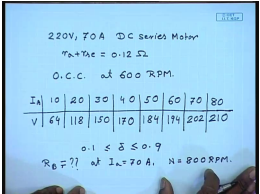For that let us assume that a DC series motor the following rating 220 volt, 70 ampere motor, the combined resistance r a plus r s e equal to 0.12 ohm, and the O C C at 600 R P M is given by the following data . 10, 63, 20, 40 ampere, this is the O C C at 600 R P M . Now, you want to break this, this is series motor using dynamic braking and the chopper. The chopper duty ratio changes from. So, what should be the value of R B. So, that at I a equal to 70 ampere. The motor and the braking region the motor speed is maximum motor speed is 800 R P M.

For that what should be the braking resistance R B. From the magnetization data we find that for N equal to for I a equal to 80 and speed equal to 60. The back unit is 210 therefore, at I a equal to same 70 ampere and back e m f and speed is equal to 800 R P M. We can find out the back e m f, N equal to 800 R P M, I a equal to 70 ampere.

(Refer Slide Time: 04:44)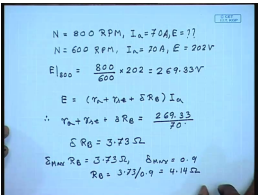First we have to find out, what is E. The given data is N equal to 600. I a equal to 70 ampere. E equal to 202 volts therefore, E at 800 will be equal to 800 by 600 into 202, 269.33 volts. So, in the regenerative braking we know that E equal to r a plus r s e plus delta R B. So, into I a term r a plus r s e plus delta R B comes to 269.33 divided by 70 or delta R B equal to 3.73 ohm. Now at maximum speed we will keep delta equal to at its maximum value. So, delta max R B equal to 3.73 ohm or delta max equal to 0.9 therefore, R B equal to 3.73 divided by 0.9 this gives me 4.14 ohm.

(Refer Slide Time: 07:54)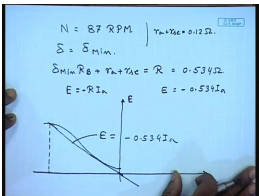Now, suppose with this braking resistance let us try to find out what is the maximum torque we can get with this chopper when the speed goes down to N equal to 87 R P M. Now as the speed goes down we also reduce the effective resistance then at lower speed we will operate at delta equal to delta min. So, the total delta min R B will be the effective resistance plus r a plus r s e will be the braking resistance R. This will come to 0.534 ohms as r a plus r s e is given as. Also E equal to R I a. So, if we plot from the given data at 600 R P M we can plot the E versus I a at 87 R P M, and then also plot E equal to this is has to be taken in the reverse direction because current has reversed minus 0.534 I a and find out the intersection point turns out. At the intersection point I a comes out to be approximately 50 ampere.

(Refer Slide Time: 10:24)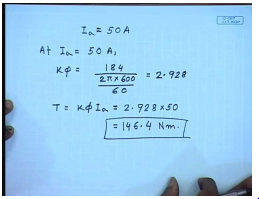So, at 50 ampere from the given data, we have E equal to 184 volts at 600 R P M. So, K phi at I a equal to 50 ampere, K phi equal to 184 volts divided by 600 2 pi into 600 by 60 this comes to 2.928 therefore, T equal to K phi I a equal to is 146.4 newton meter . So, we can still generate so much torque at a reduced speed of just 87 R P M. So, we can also apply regenerative braking and let us see during regenerative braking what kind of torque we can expect.

(Refer Slide Time: 12:27)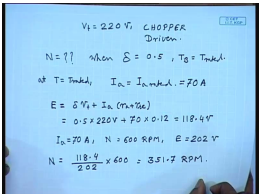So, let us say this same machine is supplied from a V t of 220 volts through a chopper and chopper driven. So, what should be the motor speed when delta equal to 0.5 and braking torque equal to T rated. Now at rated motor torque at T equal to T rated whether it is motoring operation or braking operation I a will be equal to I a rated. This is 70 ampere. So, what is E now it is braking operation. So, E equal to delta V t plus I a into r a plus r s e that is equal to 0.5 into 220 volts plus 70 into 0.12 this comes to 118.4 volts.

From the magnetization characteristics we know that at I a equal to 70 ampere and N equal to 600 R P M. E is equal to 202 volts. So, if E is to be 118.4 volts at the same armature current then the speed must be. So, even at this speed the regenerated braking torque equal to the maximum rated motor torque can be produced using a chopper.

(Refer Slide Time: 15:44)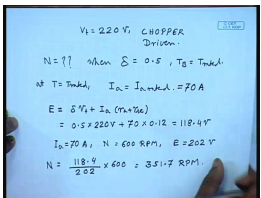Now, also let us try to find out what is the maximum motor speed if I want that I a to be same 70 ampere and if delta max is equal to 0.95 what is the maximum motor speed. At 70 ampere please note that it will still generate the rated braking torque because the current is rated . So, what is E, E equal to delta max into V plus I a into r a plus r s e 0.95 into 220 plus seventy into 0.1 two this comes to 217.4 volts.

Now, for I a equal to 70 ampere, N equal to 600 R P M, E equal to 202 volt. And I a equal to 70 ampere and E equal to 217.4 volts, then N should be equal to 217.4 by 202 into 600 equal to 645. 7 R P M. So, we see that 645. 7 R P M we have a armature current of 70 ampere. The duty cycle of 0.95 the motor produces rated braking torque. also at 351 R P M we can reduce delta to 0.5 and still obtain rated regenerative braking torque. In fact, delta can be reduced further then even at a lower speed equal amount of regenerative braking torque can be obtained by using a chopper. In fact, choppers can be used for speed control of DC series motor.

(Refer Slide Time: 18:31)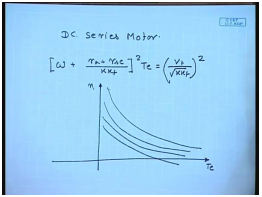So, you know for DC series motor, the torque speed characteristics is given by. In order to this torque speed characteristics looks for constant r a and V t its looks like this. there are two ways to change this, one is to change the armature resistance with increasing armature resistance I can reduce the lower the torque speed characteristics; however, we as we know this is involves lot of loss in the additional armature resistance. Another more efficient method will be to control V t and this V t can be controlled by using a chopper.

(Refer Slide Time: 20:08)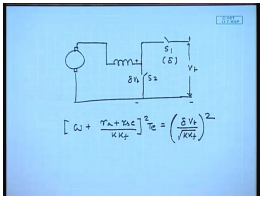So, if we fit the DC series motor from a chopper, and this is one operates at the duty ratio of delta then the terminal voltage here will be delta V t and the torque speed characteristics will be given by… Hence by changing delta this is increasing delta.

(Refer Slide Time: 21:32)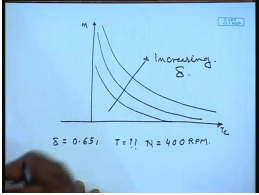So, let us see through a problem the quantitative effect the same motor that we have been using now let us try to find out, if I set a delta equal to 0.65 then what should be the torque for N equal to 400 RPM.

(Refer Slide Time: 22:58)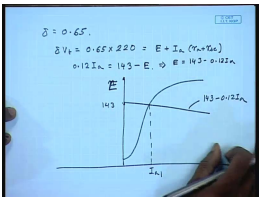So, with delta equal to 0.65 delta V t equal to 0.65 into 220, and this should be equal to E plus I a, r a plus r s e or 0.12 I a equal to 143 minus E . So, we can find out where this intersection point will be, at N equal to 400 RPM. We are given the O C C at 600 RPM. These will we can scale to 400 RPM and on this we can plot. So this is 143 this will be intersection point I a 1. This I a 1 this is 143 minus 0.12 I a, this gives I a approximately equal to 70 amperes.

(Refer Slide Time: 25:12)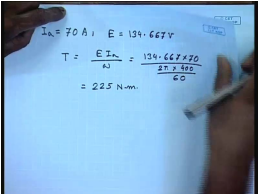And also gives a E of 134.667 volts. Torque will be equal to E I a by omega that is one 134.667 into 70 RPM. Please remember this should be O C C at 400 RPM not 600 RPM. 2 pi into 400 by 60 this comes to 225 Newton meter. So, given the delta and speed we can find out the torque or if we given the delta or current we can find out the speed. So, chopper control is an effective method of controlling the speed of a DC series motor efficiently.

However if we do not this has to be remembered that this chopper which is connected to the machine terminal has to carry the whole machine current. So, the switches that are used in this chopper should be designed to withstand the full current of the machine this makes the system of speed controls somewhat expensive there are other simpler method of controlling the speed of DC series motor in which case we can see that we can also control the value of k k f in the equation of torque speed characteristics in order to control the speed of a DC series motor. There are several methods for doing this one is called the field diverter control.

(Refer Slide Time: 28:02)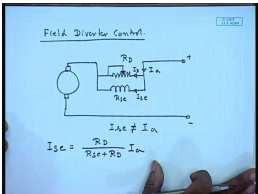In this what we do is that, the field is shunted by a variable diverter resistance R d. If this is R s e and the total current is I a then there in there is current I d and I s e. So, once you place a diverter by adjusting the value of R d we can adjust the value of I s e, and I s e in this case will not be is not equal to I a. We can also find out what will be the value of I s e. I s e equal to R d divided by R s e plus R d into I a. For in which case the torque speed characteristics will also change because E equal to K phi I a equal to K K f, I s e, I a.

(Refer Slide Time: 30:08)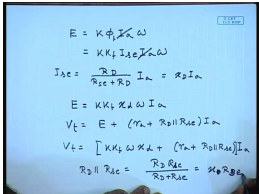Now, we have I s e equal to R d divided by R s e plus R d into I a. Let us call it x d I a therefore, E equal to K K f x d , this is omega. omega I a V t equal to E plus now r a plus R d parallel R s e into I a. So, V t equal to… Now R d parallel R s e is equal to R d, R s e divided by R d plus R s e this is equal to x d R d or rather x d R s e.

(Refer Slide Time: 32:52)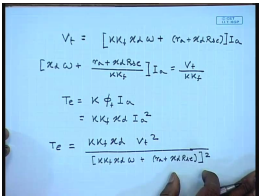Therefore V t equal to, so current speed characteristics now will be … And the torque speed characteristics will be T e equal to K phi f I a equal to K K f x d I a square T e equal to …

(Refer Slide Time: 36:05)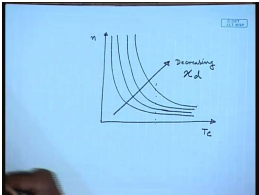We draw these torque speed characteristics it will look somewhat like, this is decreasing x d that is this amounts to weakening the series field. So, we know that for the same torque if the field is weakened the speed increases. There are another method on somewhat the same line is called the tapered field control, in which tapped field control, in which there are several taps.

(Refer Slide Time: 37:23)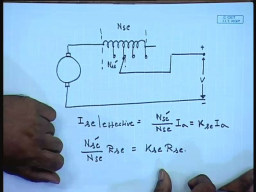There are several taps that are taken from the series field, and actually at any given moment the field can be connected to any one of those taps. Here also if we are basically changing the field ampere turns and hence the field flux. Let us say the total number of series field terms is N s e and between the present tap it is N s e dash. So, effective series current I s e equal to N s e dash divided by N s e into I a which is some k s e I a . So, the resistance also next will be somewhat assuming them to be proportional to n s e dash by N s e into R s e to K s e R s e. As in this case also we will get similar torque speed characteristics as in the case of field diverter where x d will now be replaced by R s e. A special case of this type of field control is called where the field winding is arranged is in several sections and series parallel combination of these sections are used.

(Refer Slide Time: 40:00)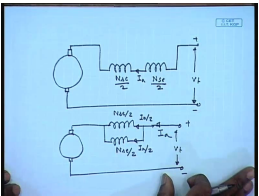For example let us say that we have a DC series machine whose field winding has two sections of equal number of turns, each N s e by 2. Now these two series these two field winding can also be connected in parallel like this, whereas in this case the total current flowing to each of them is I a. Here it is I a by 2 and this is I a by 2 total current being same I a.

(Refer Slide Time: 41:39)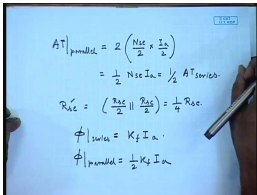Then A T ampere turns of the series field winding with parallel connection is A T with parallel connection will be equal to two times the A T of individual winding, which is N s e by 2 into I a by 2 this is equal to half N s e I a, which is half of A T with series connection. Also R s e dash will be R s e by two parallel R s e by two is equal to one fourth of R s e. So, K s e in this case, we assume linear field then phi in series will be equal to will be K f I a whereas, phi with parallel connection will be equal to half K f I a. Hence only two speeds are possible, here only two speeds are possible. So, let us try to solve the how a few problems when how a speed control can be achieved.

(Refer Slide Time: 44:23)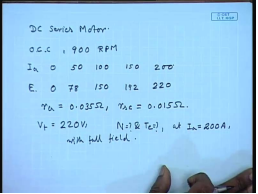Let us take a DC series motor, the O C C is given by I a e 0, 0, 50 ampere, 70, 800 ampere. This is at 900 RPM, 150 volts, 150 ampere, 192 volts and 200 ampere, 220 volts. The r a equal to 0.035 ohm and R s e equal to 0.015 ohm the supply voltage v t equal to 220 volts. What should be the speed and torque of the motor if at I a equal to 200 ampere with full field.

(Refer Slide Time: 46:56)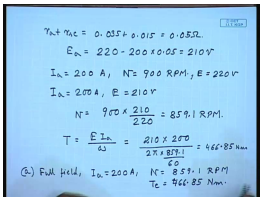Let us first find this out with field total r a plus R s e equal to 0.035 plus 0.015 equal to 0.05 ohm. E a equal to 22 minus 200 into 0.05 equal to 210 volts. So, with with I a equal to 200 ampere. N equal to 900 RPM we know e equal to 220 volts. Now, here are same I a equal to 200 ampere, we have got an E of 210 volts. So, N equal to 900 into 210 divided by 220 this comes to 859.1 RPM. So, what is T e I a by omega. So, 210 into 200 divided by 2 pi into 859.1 by 60. This comes to 46.85 Newton meter . So, with full field at I a equal to 200 amperes we got N equal to 859.1 RPM and T e equal to 466.85 Newton meter.

(Refer Slide Time: 49:49)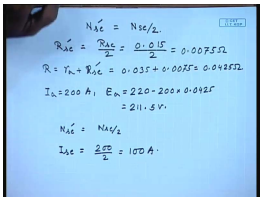Now, let us see how these values will change when the field is field turns are reduced to half. Then the resistance R s e dash will be equal to R s e by 2, that will be 0.01 and 0.5 by 2, 0.0075 ohm. So, total resistance R equal to r a plus R s e dash will be 0.035 plus 0.0075. This will be equal to 0.0425 ohm. So, the when I a equal to 200 ampere then E a will be 220 minus 200 into 0.425 equal to 211.5 volts. Now, N s e dash equal to N s e by 2. So, effective field current effective full field current equal to I s e equal to 200 by two equal to 100 amperes.

(Refer Slide Time: 52:31)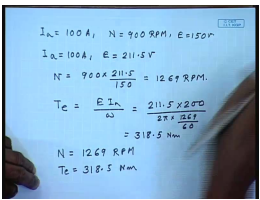So, for 100 amperes, we have the given data we have seen that at I a equal to 100 amperes, and N equal to 900 RPM. We have got E equal to 150 volts therefore, now if our we have same I a equal to 100 amperes, and E equal to 211.5volts therefore, N will be 900 into 211.5 divided by 150 equal to 1269 RPM. And torque will be E I a by omega that is 211.5, but armature current is 200 ampere divided by 2 pi into 1269 by 60.

This will come to 318.5 Newton meter. So, with half the series field winding now we have N equal to 1269 RPM, and T e equal to 318.5 Newton meter. comparing with the previous result we find that as the series field turn reduces the speed increases from 859 to 1269, and the torque decreases from 466 to 318. This is the field weakening mode of operation of DC series motor.

Thank you.

Offer running on EduRev: Apply code STAYHOME200 to get INR 200 off on our premium plan EduRev Infinity!

61 docs|20 tests

,

,

,

,

,

,

,

,

,

,

,

,

,

,

,

,

,

,

,

,

,

;